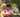### Passing Cars

January 17, 2020

Passing Cars

A non-empty array A consisting of N integers is given. The consecutive elements of array A represent consecutive cars on a road.

Array A contains only 0s and/or 1s:
• 0 represents a car traveling east,
• 1 represents a car traveling west.

The goal is to count passing cars. We say that a pair of cars (P, Q), where 0 ≤ P < Q < N, is passing when P is traveling to the east and Q is traveling to the west.

For example, consider array A such that:
A = 0
A = 1
A = 0
A = 1
A = 1
We have five pairs of passing cars: (0, 1), (0, 3), (0, 4), (2, 3), (2, 4).

Write a function:
function solution(A);
that, given a non-empty array A of N integers, returns the number of pairs of passing cars.
The function should return −1 if the number of pairs of passing cars exceeds 1,000,000,000.

For example, given:
A = 0
A = 1
A = 0
A = 1
A = 1
the function should return 5, as explained above.

Write an efficient algorithm for the following assumptions:
• N is an integer within the range [1..100,000];
• each element of array A is an integer that can have one of the following values: 0, 1.

0 ≤ P < Q < N : the zeros will show before ones
Add the ones count from the end of the array, and add the previous ones count for each passing zero.

``````function solution(A) {
// write your code in JavaScript (Node.js 8.9.4)
// P < Q, P -east, 0; Q -west, 1
// the index of 0 shows before 1
// counting 1 from the end, add sub-total for each 0

var ones = 0, passing = 0;
for(var i=A.length-1; i>=0; i--) {
//console.log("i: ", i, " passing: ", passing, " ones: ", ones)
if (A[i] === 0){
passing += ones;
if (passing > 1000000000){
return -1;
}
} else {
ones ++;
}
}
return passing;
}``````Posted by Tai Lu ( 呂台生 ) who's building useful things.You should follow him on Twitter